# Construction Calculator A1 Pro v2.5.6 build 24 APK [Paid] [Latest]

LegendAPK, Thursday, January 12, 2023Requirements: 4.1 and up
Overview: Construction Calculator A1 pro is a paid android application for construction calculations

Construction Calculator A1 pro can calculate with the Imperial Measurement System & Metric Measurement system.

App also supports a number of themes choose the color you want.

Construction Calculator A1 pro is a paid android application for construction calculations. We use simple tools in the application to simplify the calculations for the construction industry. We help to calculate almost all kinds of area, estimation calculation, volume calculation, unit converters, and normal calculator as well.

We have divided the application into some parts like quantity calculator, area calculator, volume calculator, unit converter, and normal calculator.

This calculator is useful for Civil engineers, Site Supervisors, Civil Engineering students, Mechanical Engineers, Construction Project Managers, Construction Store managers, Fresher Engineers, Construction Contractors, Building Contractors, Storekeepers, Site Execution engineers, Estimation engineers, and many more. Even a normal person who needs to do basic home calculations then he also needs this app. You can calculate the plot area so it will be useful for Real estate agents as well.

Quantity Estimation App For Construction
Quantity Calculation of Building Materials
Building calculator is divided into the following four categories:-

Quantity Calculator includes-
-Reinforcement Steel Calculator
-Steel weight Calculator
-Concrete Calculator(with Volume, without volume, Circular Column)
-Excavation Calculator
-Backfill Calculator
-Brickwork Calculator
-Tile Calculator
-Plaster Calculator
-Paint
-Water Tank Capacity Calculation(Circular and Rectangular)
– Material Density
-AC Capacity calculator
-Swimming pool
-Solar(Electric)
-Solar water heater
-Plywood calculator
-Paver calculator
-Plum concrete
-Rainwater harvesting
-Waterproofing material calculator
-Shuttering calculator
-Grout calculator
-Other Quantities and many more

Area Calculator includes-
-Area Measurement App
-Area Calculator for Land
-Circle Area Calculator
-Rectangle Area Calculator
-Triangle Area Calculator
-Rhombus Area Calculator
-L plot Area Calculator
-Square Area Calculator
-Right Angle Area Calculator
-Sector area calculator
-Pentagon area calculator
-Hexagon area calculator
-Octagon calculator
-Trapezoid Area calculator
-Other areas and Many more

Volume Calculator includes-
-Sphere Volume Calculator
-Cube Volume Calculator
-Block Volume Calculator
-Bucket Volume Calculator
-Semi Sphere Volume Calculator
-Cone Volume Calculator
-Cylinder Volume Calculator
-Trapezoid Volume Calculator
-Rectangular Prism Volume Calculator
-Spherical Cap Volume Calculator
-Frustrum Volume Calculator
-Hollow Rectangle Volume Calculator
-Tube Volume Calculator
-Slope volume calculator
-Parallelepiped volume calculator
-Sliced cylinder volume
-Barrel volume calculator
-Other Volume Calculator and many more

Unit Convertor includes-
-Length
-Weight
-Area
-Volume
-Temperature
-Pressure
-Time
-Speed
-Fuel
-Angle
-Force
-Power
-Density
-Fraction to decimal
-Number to word

***Features available only for pro version are-
-Steel Weight calculator
-Steel footing calculator
-Steel column calculator
-Steel beam calculator
-Steel slab calculator
-Concrete Tube
-Concrete of Gutter
-Concrete of Shear Wall
-Construction Cost
-AAC/CLC Block
-Asphalt
-Anti Termite
-Gypsum/POP Plaster

This app features-
-Easy and simple to use
-Added Default standard values for Simplification
-Easy interface for everyone
-A non-technical person can use
-Gives accurate information
-Fast in Calculation
-Almost all construction calculations included
-Can reset data if an error in entry
-Scientific Calculator is included(Credits: Num-Plus-Plus by DylanXie123)

We appreciate all the feedback from your side. Your suggestions and advice will help us to improve our app. If you have any suggestions about the application then feel free to contact us by email constructioncalculatorallinone@gmail.com

★ Untouched Paid apk with Original Hash Signature
★ Certificate MD5 digest7732bec273d241f42a66a9eedc46b232
★ No changes were applied
➡ Languages: Full Multi Languages
➡ CPU architectures: arm64-v8a, armeabi-v7a
➡ Screen DPIs: 120dpi, 160dpi, 240dpi, 320dpi, 480dpi, 640dpi

What’s New:

No changelog

Screenshots

•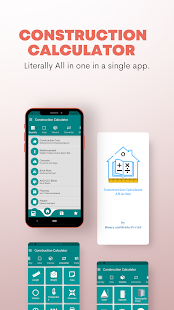••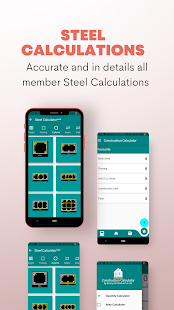••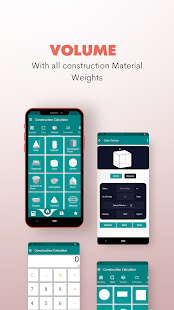••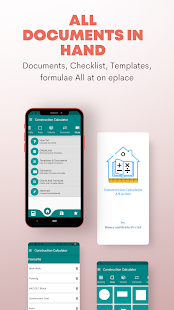•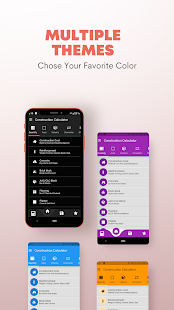Construction Calculator A1 Pro v2.5.6 build 24 APK [Paid] Armeabi-v7a / Mirror

Construction Calculator A1 Pro v2.5.6 build 24 APK [Paid] Arm64-v8a / Mirror

Older Version

Construction Calculator A1 Pro v2.5.3 build 19 APK [Paid] Armeabi-v7a / Mirror

Construction Calculator A1 Pro v2.5.3 build 19 APK [Paid] Arm64-v8a / Mirror

Construction Calculator A1 Pro v2.5.3 APK [Paid] Armeabi-v7a / Mirror

Construction Calculator A1 Pro v2.5.3 APK [Paid] Arm64-v8a / Mirror